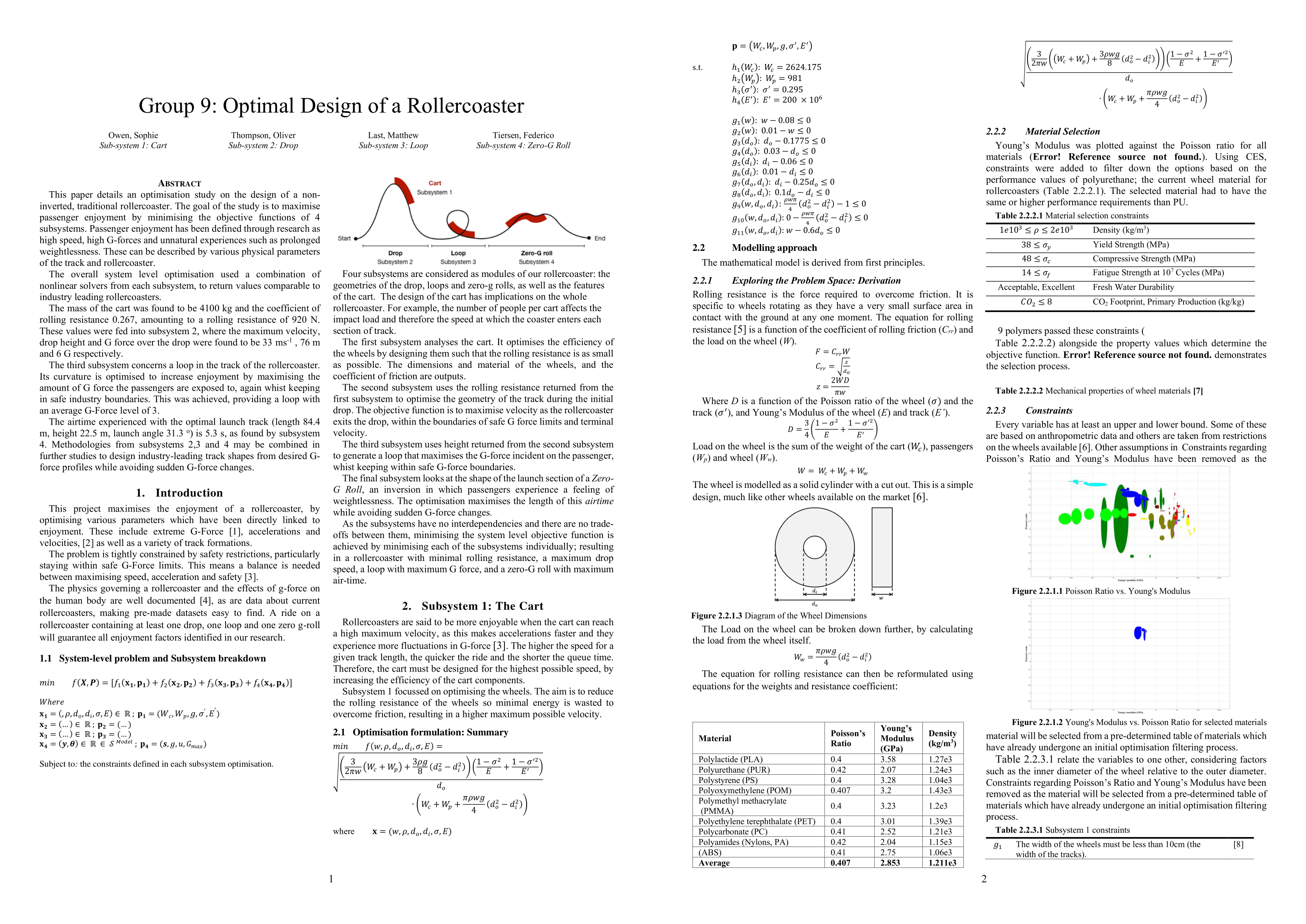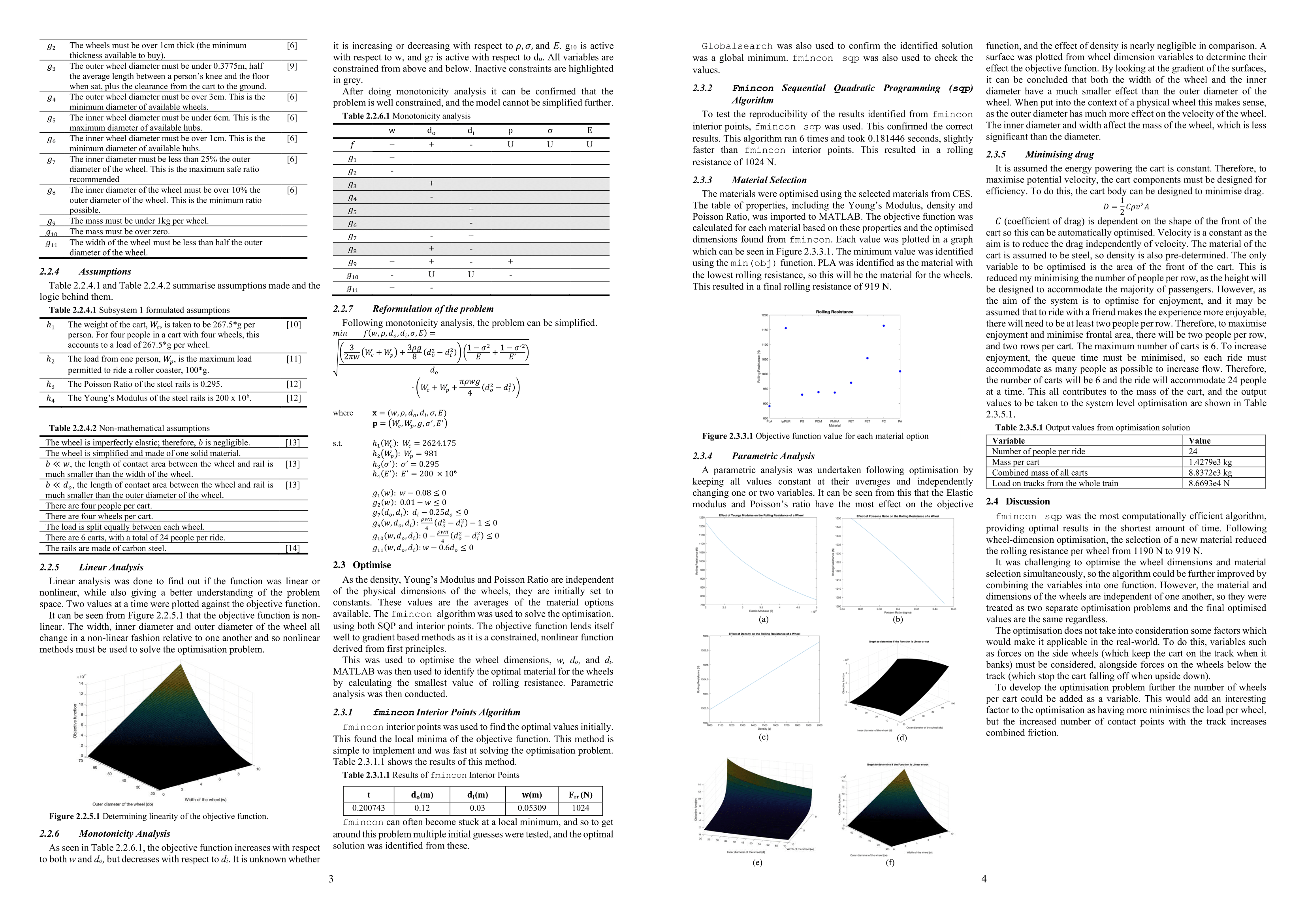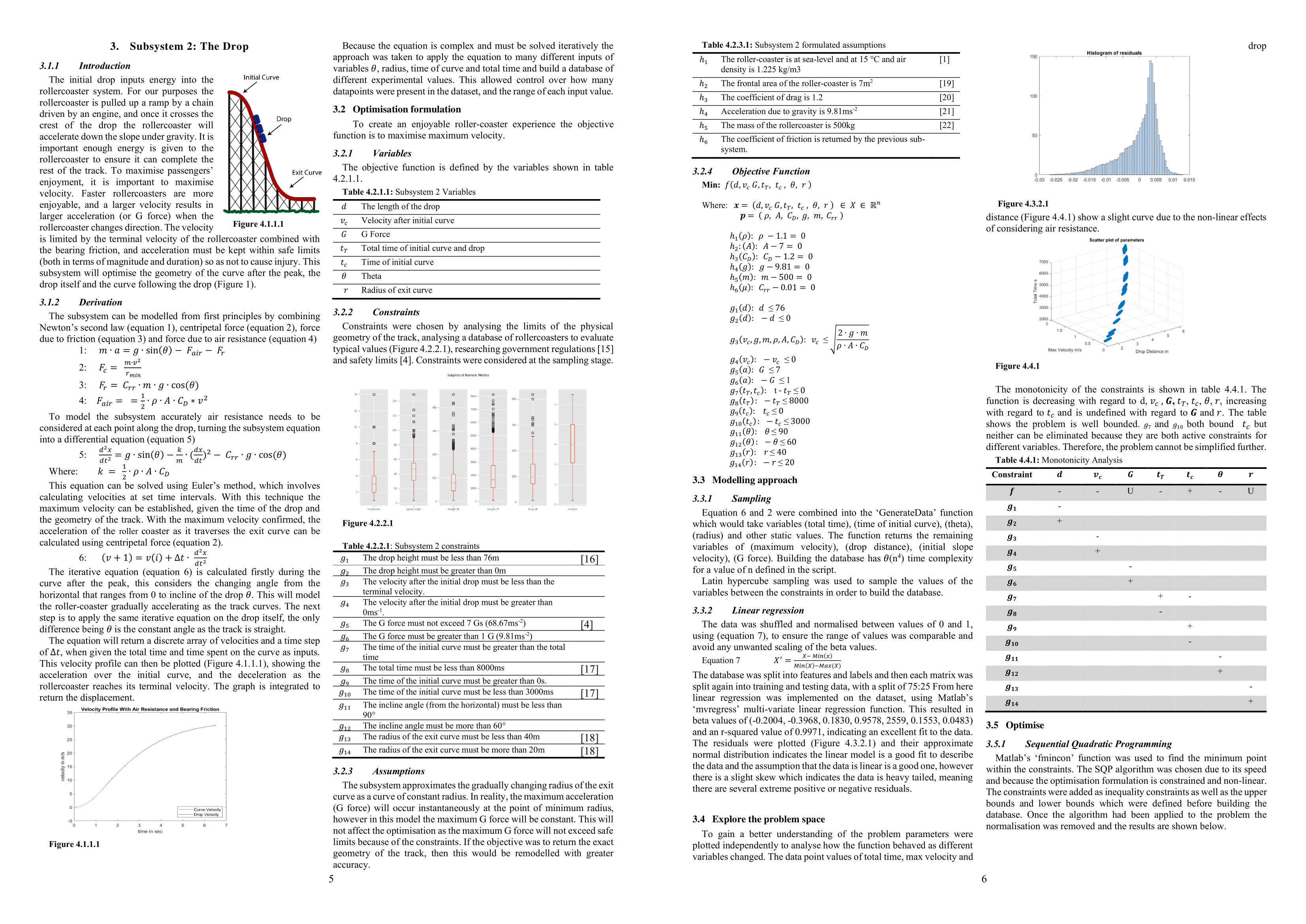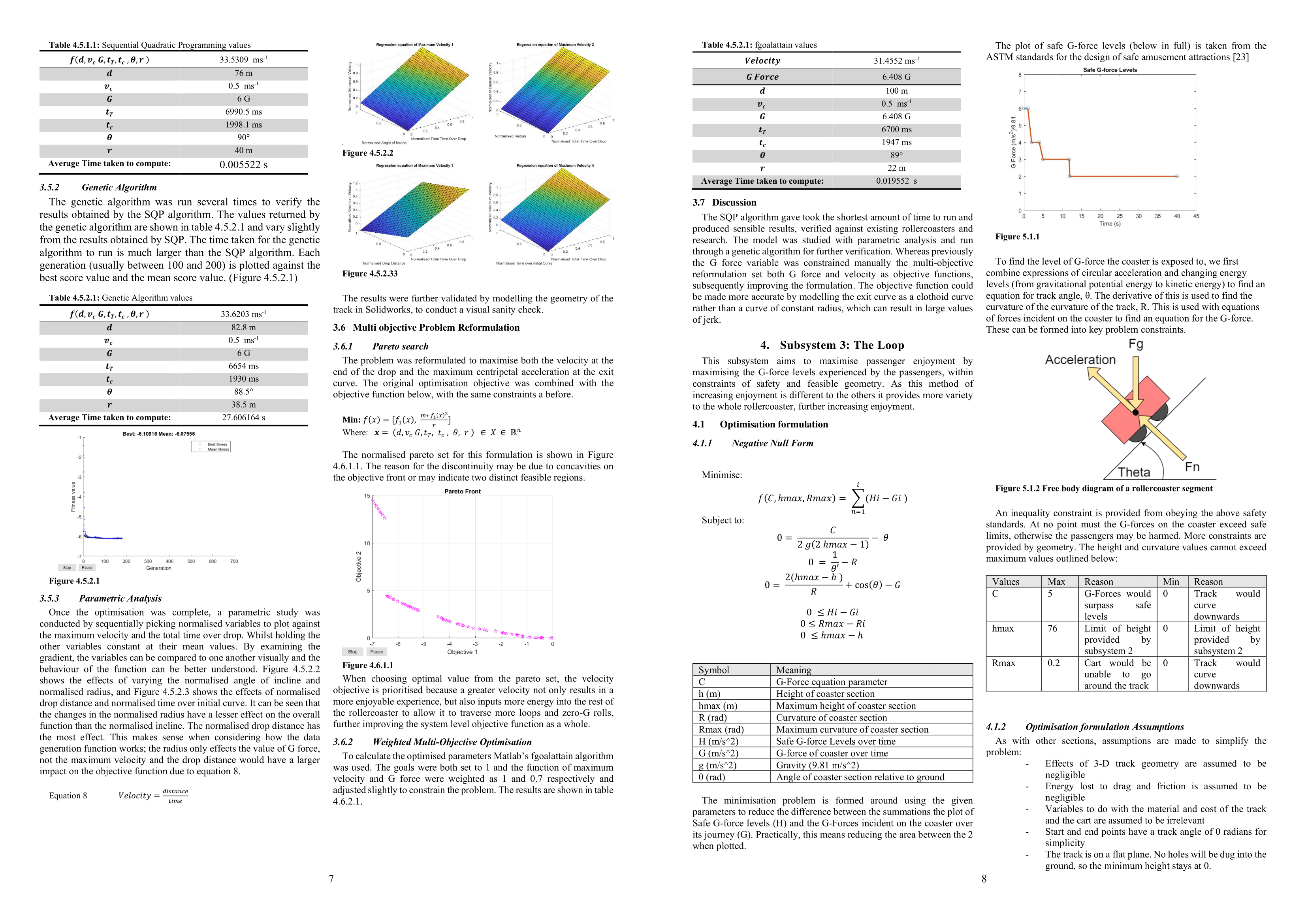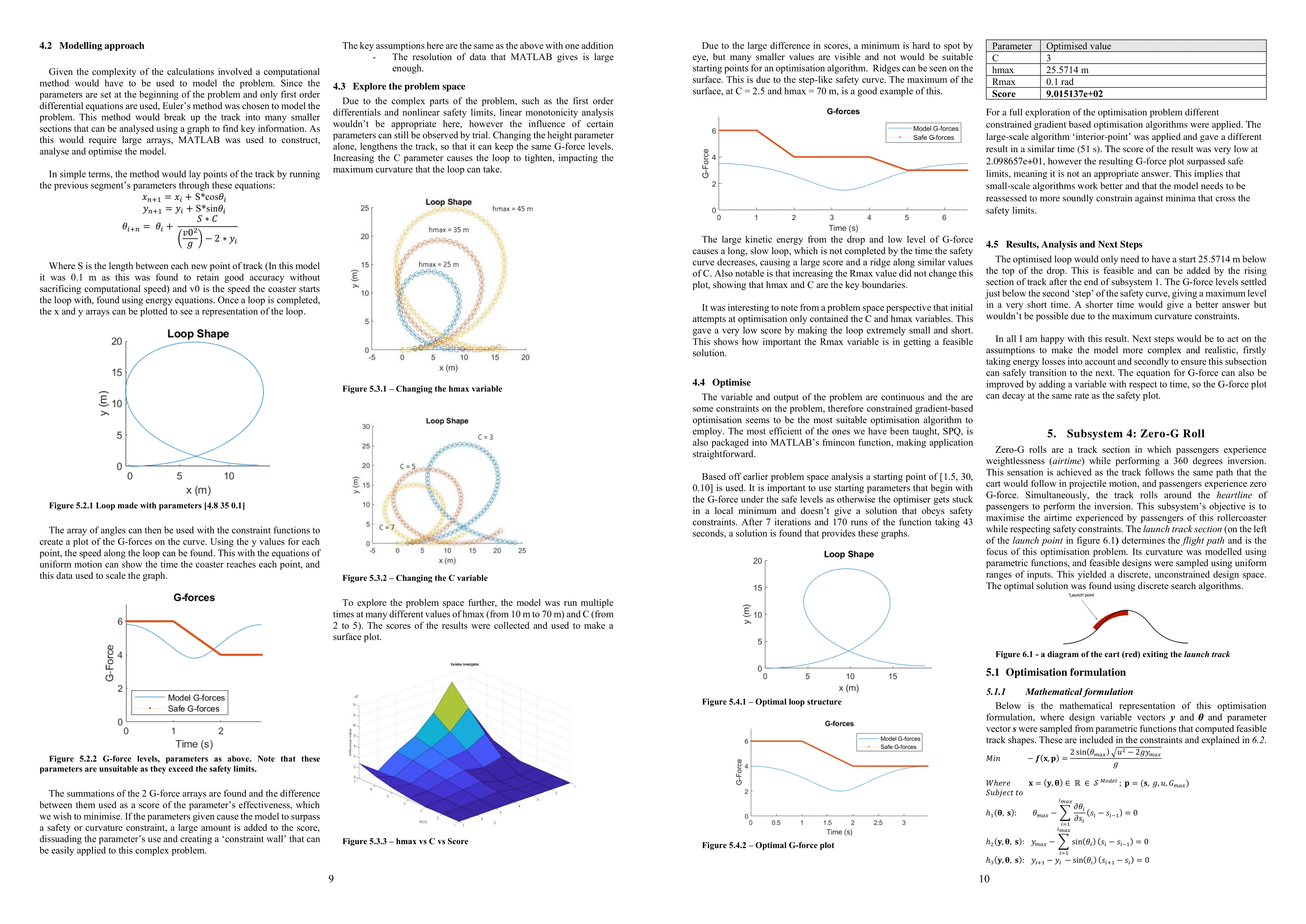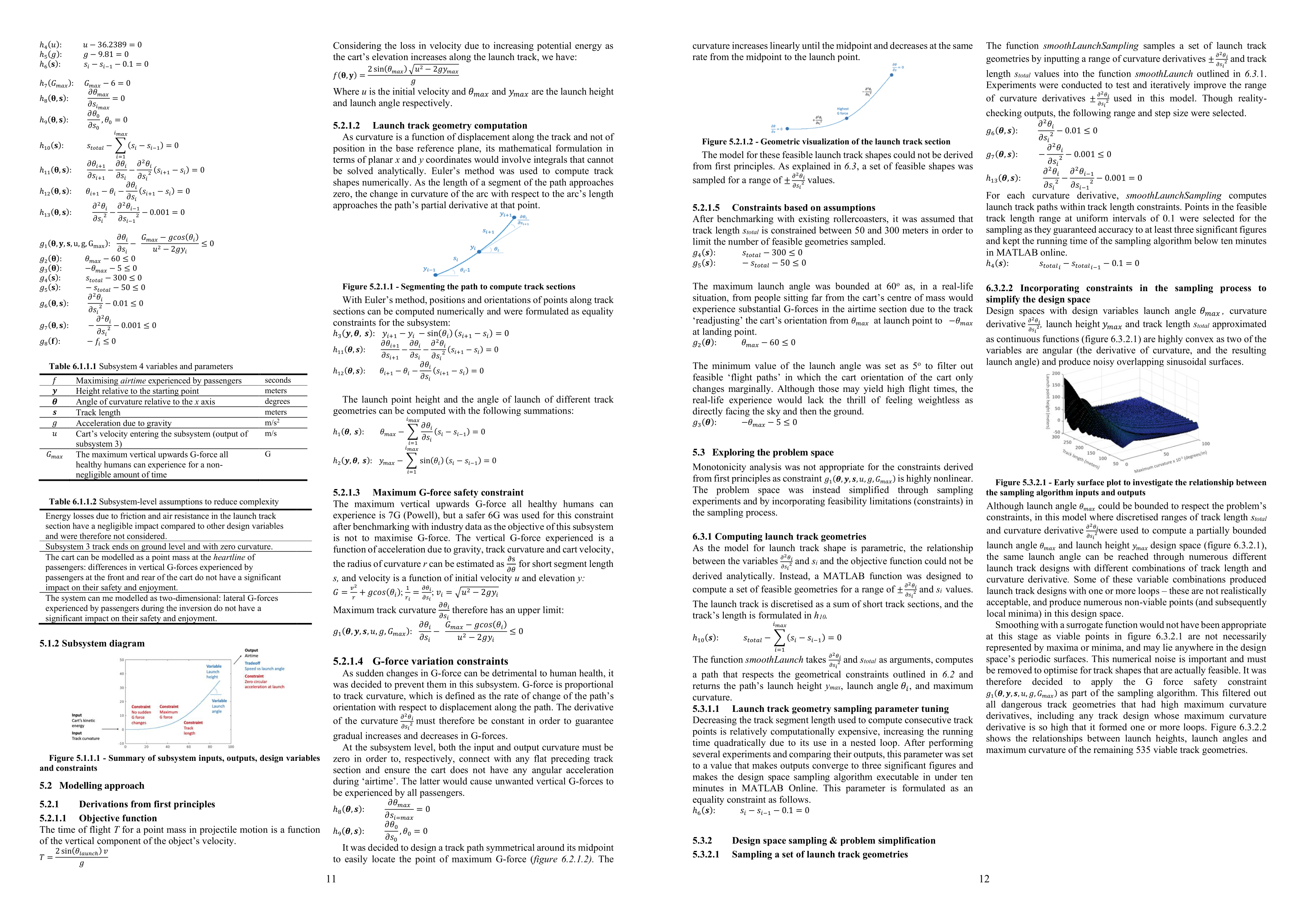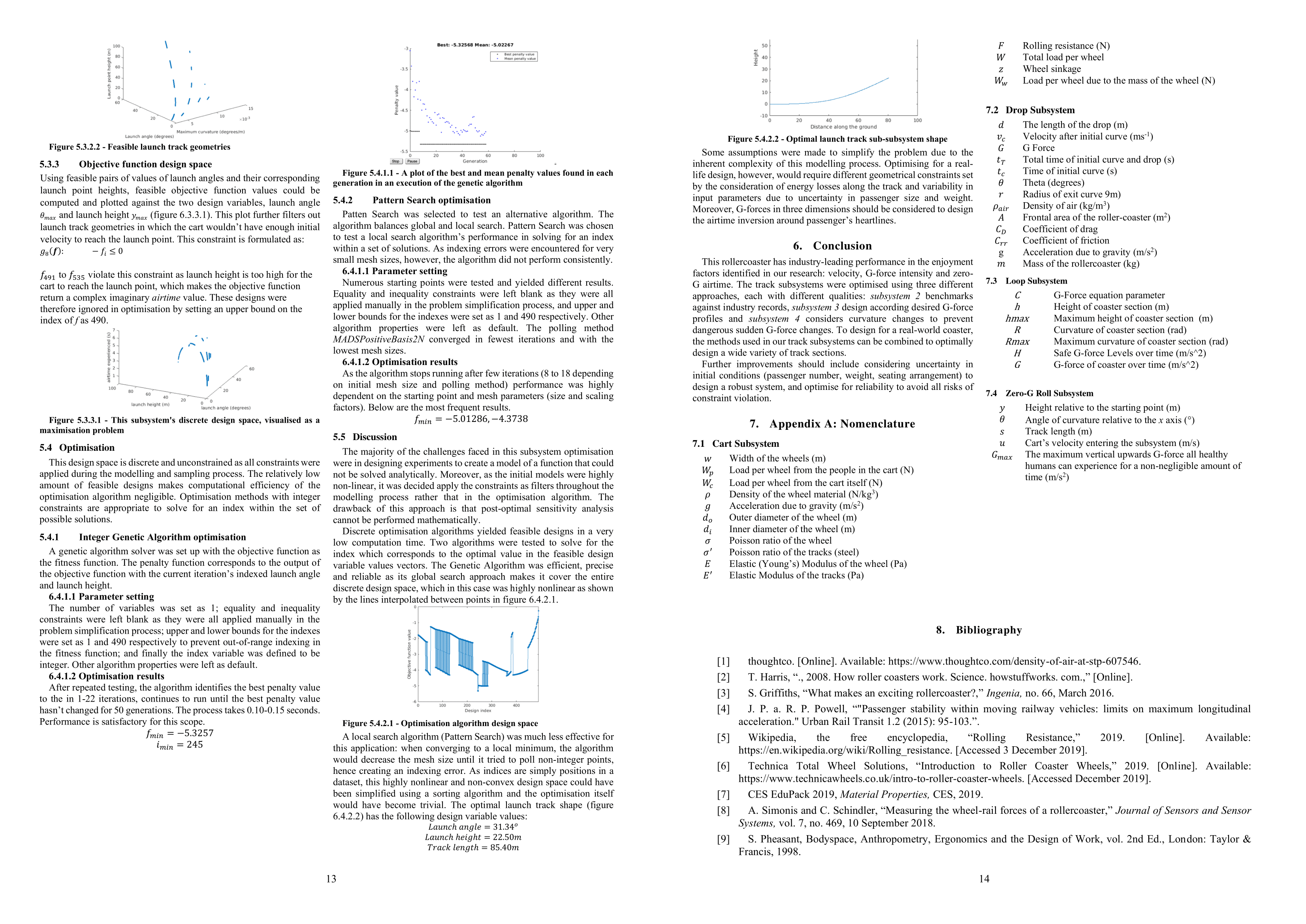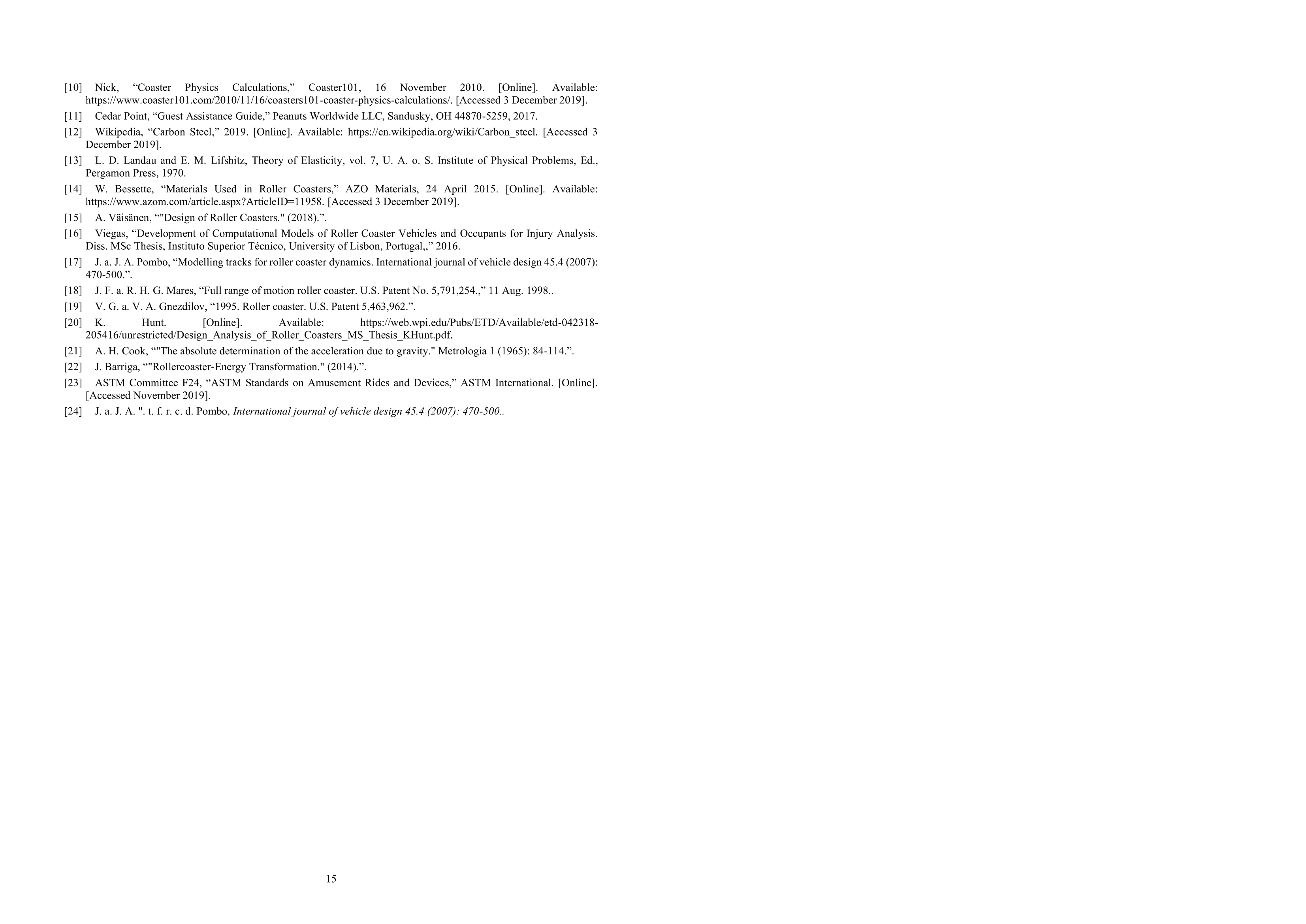# Rollercoaster Optimisation

## Matlab

• My group of 4 conducted an optimsation study on the design of a rollercoaster.
• Firstly I applied logistic regression to a rollercoaster database scraped from the web using Python.
• I then developed a multiobjective formulation from first principles, used design of experiments and implemented various optimisation algorithms to improve the design.

## Web Scraping

Before the optimisation study I conducted analytical research in support of the main project; I analysed the html of www.RCDB.com and appended the characteristics of each rollercoaster to a database and used box plots to visualise the data. Full code for this project is available on my Github.

```def scrapedata(retrievals,df):
for i in range(retrievals):   #refresh page to retrieve random rollercoaster
url = 'https://rcdb.com/'     #open up connection and grab the page
client = urq(url)
client.close()
html = bs(html, "html.parser") #parse html
rcc_text = html.find(id="rrc_text")
try: #try to find HTML tag
name = rcc_text.find("span", text=re.compile(r"Roller Coaster")).findParent("p").a.string
except:
name = None```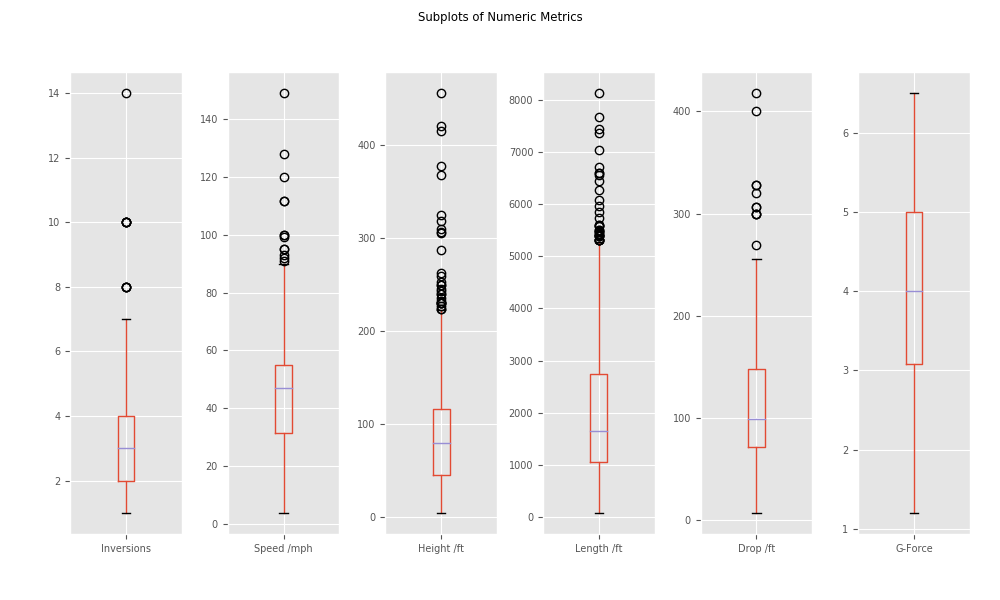## Logistic Regression

I used Python's Pandas and SKlearn to build a logistic regression classifier. I measured the accuracy of the model by plotting the confusion matrix and the ROC curve. The code allows the user to input test values into the model, which will predict wether the rollercoaster will remain operational or become defunct.

```df_temp = pd.DataFrame(x_scaled, columns=column_names_to_normalise, index = df.index)
df[column_names_to_normalise] = df_temp
X = df[column_names_to_normalise] #Define features (X) and labels (Y)
Y = df['Status']
X_train,X_test,y_train,y_test=train_test_split(X,Y,test_size=0.25,random_state=42) #split data into training and testing
logreg = LogisticRegression() # fit the model with data
logreg.fit(X_train,y_train)
y_pred=logreg.predict(X_test)
test=logreg.predict(pd.DataFrame({"Inversions": [input_inversions],"Speed /mph": [input_speed], "Height/ ft": [input_height], "Drop /ft": [input_drop] ,"Length /ft": [input_length] ,"G-Force": [input_g_force]}))
```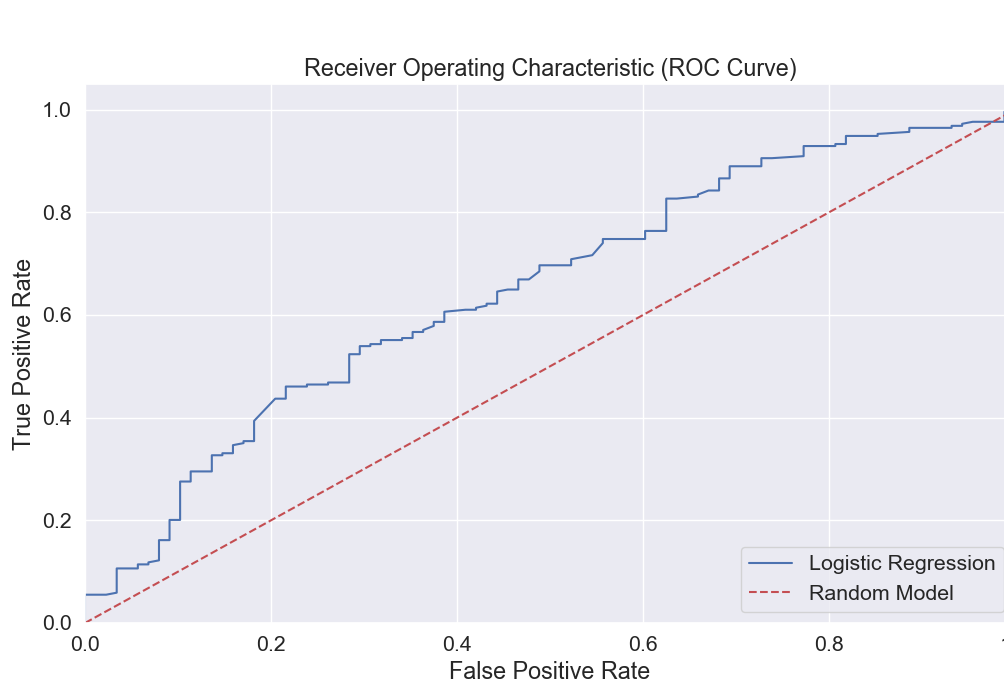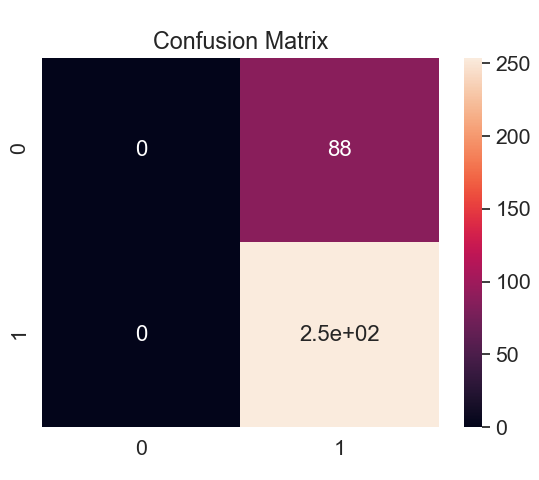## Design of Experiments

I was examining the geometry of the drop section of the rollercoaster and I derived the equations from first principles. Given that air resistance was considered the system of equations could not be solved analytically. I therefore used Matlab to build a dataset of physical values to fit a linear regression model to. The equations are implemented in the generate data function below, which is run several thousand times for different values.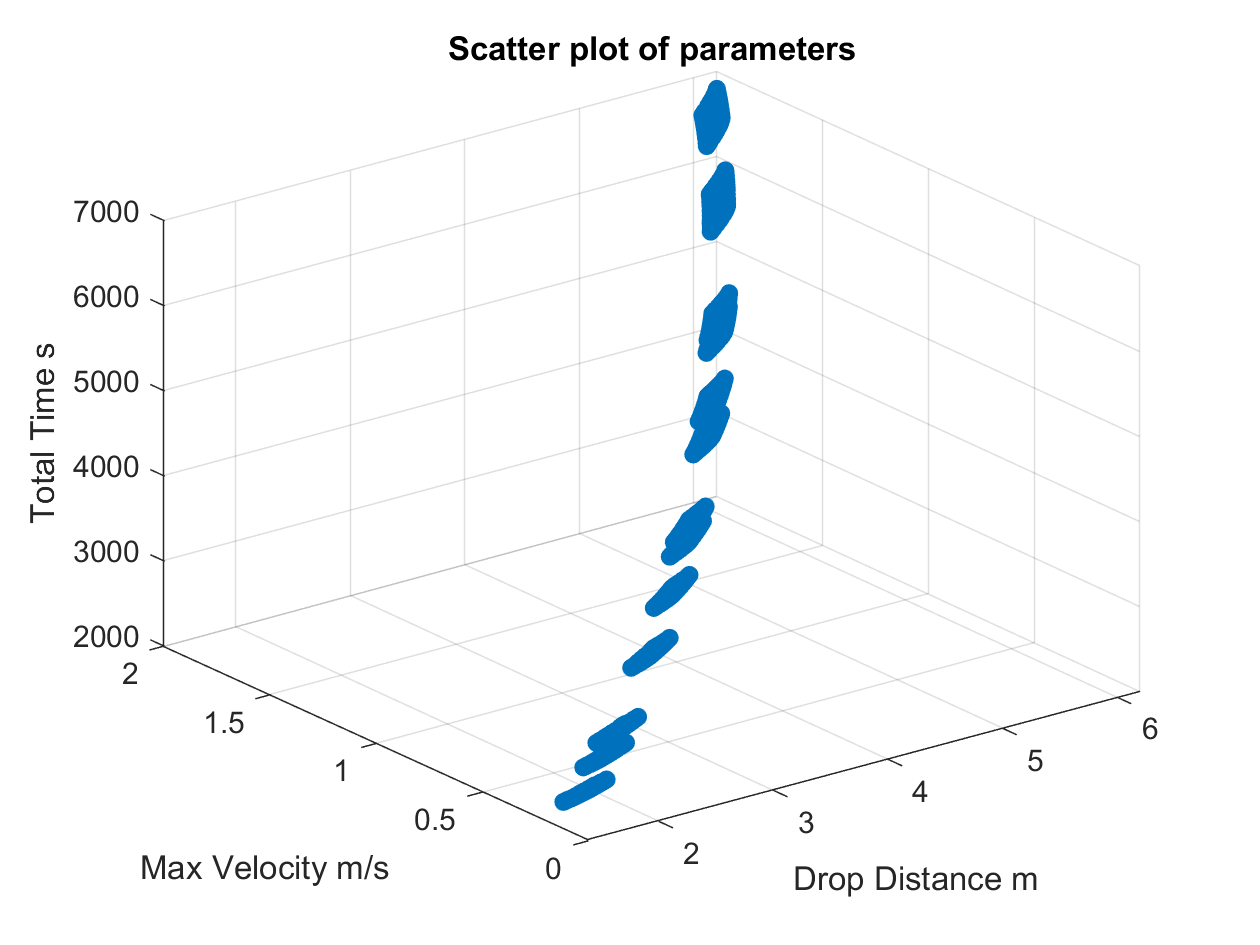```function [max_velocity, drop_distance, start_slope_velocity, g_force] = GenerateData(total_time, time_of_top_curve, theta, radius, rho, A, CD, g, mass, mu, flag)
for i=ceil(time_of_top_curve):ceil(total_time) //
V(i+1) = V(i) + dt * (g * cosd(theta) - ((k/mass) * V(i)^2) - (mu * g * sind(theta)));
S(i) = V(i)*dt;
t=(0:total_time)*dt;
vterminal=sqrt(g*mass/k); % Terminal velocity
drop_distance = sum(S);
max_velocity = V(ceil(total_time));
start_slope_velocity = V(ceil(time_of_top_curve));```

I used Latin Hyoercube Sampling to efficiently choose a subset of data points to reduce computational complexity. I iteratively applied the sample arrays to the generate data function to return the other 4 variables for the linear regression model.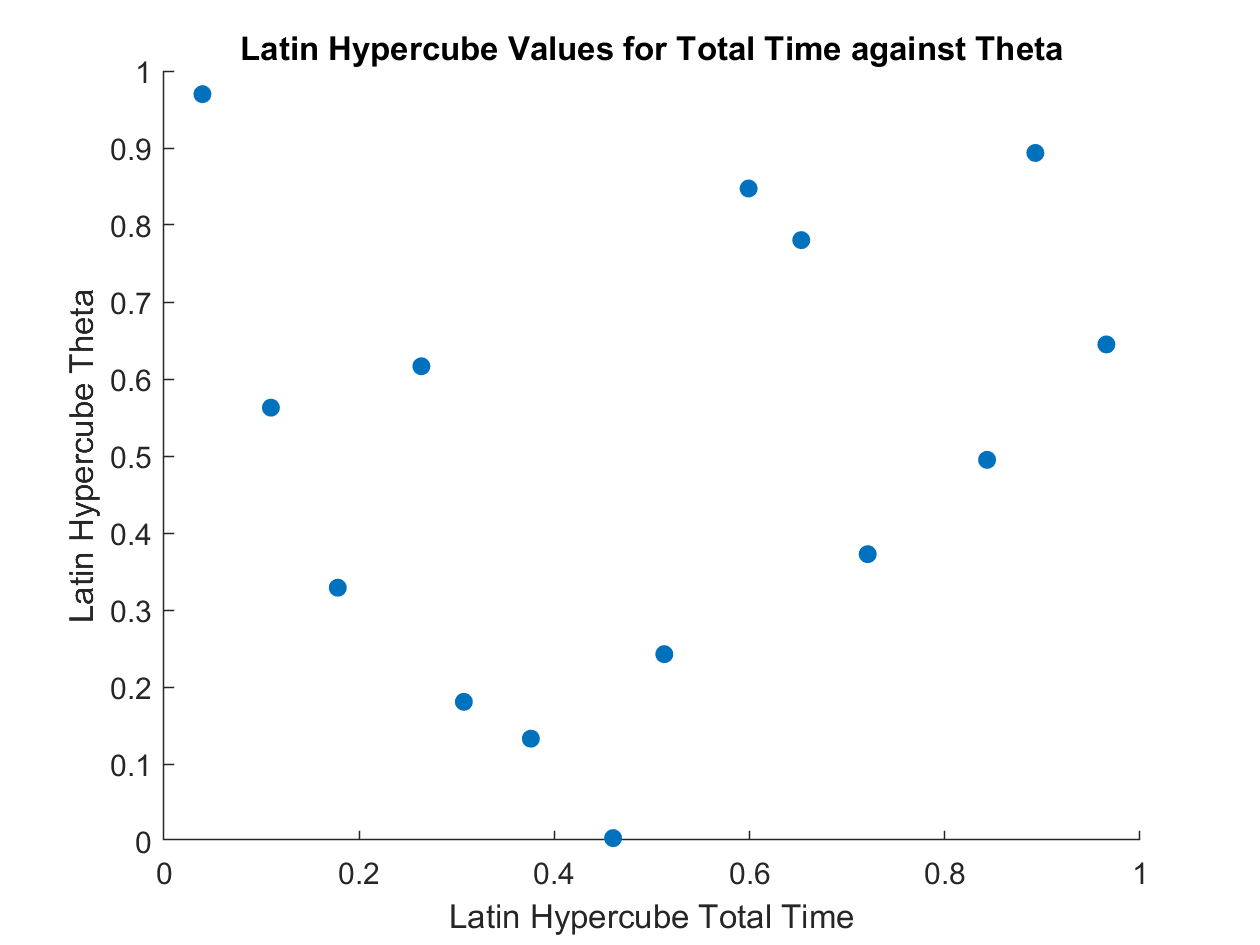```for i = 1:points
for ii = 1:points
for iii = 1:points
for iiii = 1:points
%call eulers method function that calculates remaining variables from the giveninputs
[max_velocity, drop_distance, start_slope_velocity, g_force] = GenerateData(lhs_total_time(i), lhs_time_of_top_curve(ii), lhs_theta(iii), lhs_radius(iiii), air_density, frontal_area, drag_coefficient, g, mass, friction_coefficient, false);
%append new value to database
data_base(count,:) = [max_velocity, drop_distance, start_slope_velocity, g_force, lhs_total_time(i), lhs_time_of_top_curve(ii), lhs_theta(iii), lhs_radius(iiii)];
count= count+1;
points^4;```

## Linear Regression

I normalised and shuffled the data before using Matlab's multivariate linear regression function to plot a function to the data. I also computed the R squared value and plotted the residuals.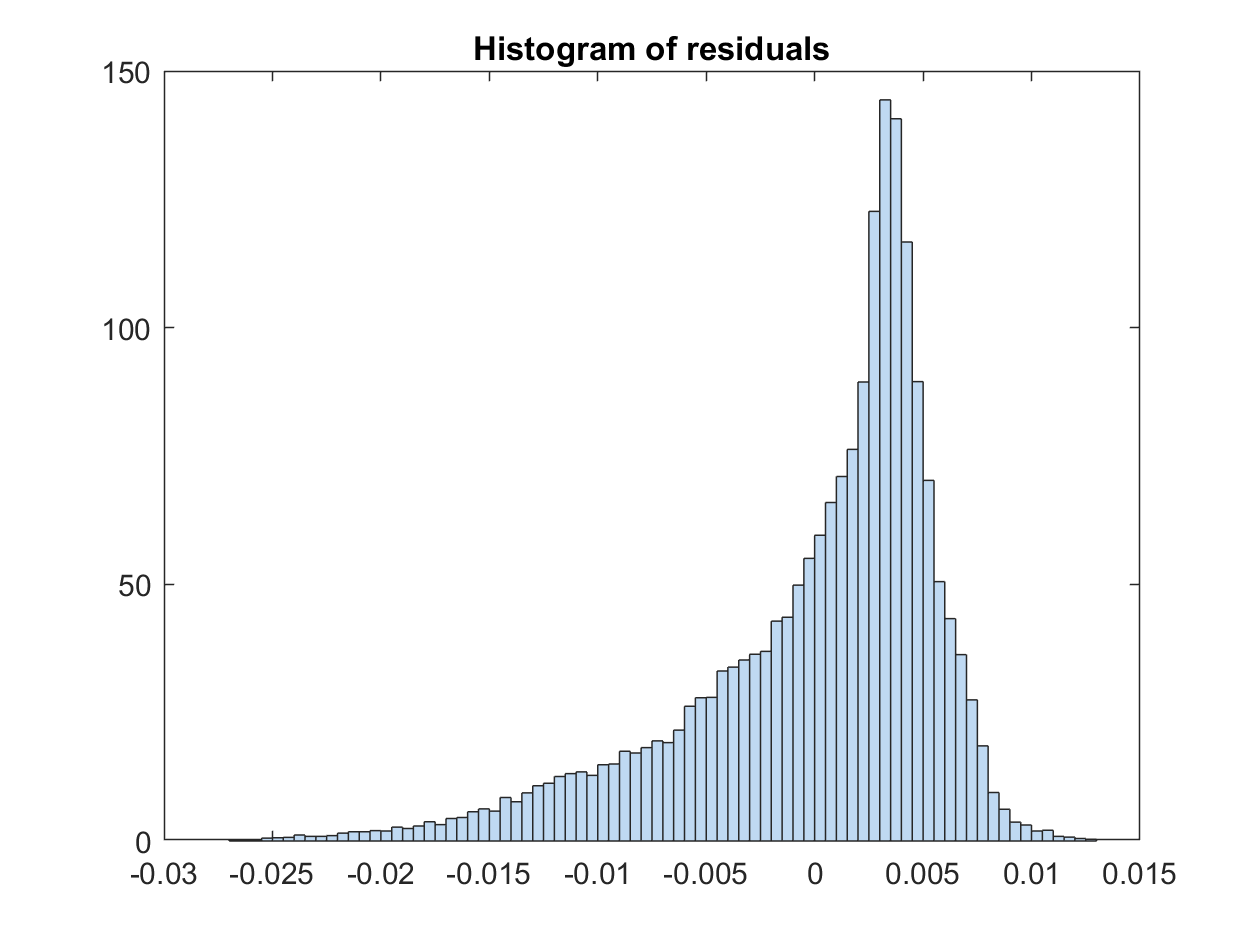```newInd = randperm(length(data_base_norm));
data_base_norm_shuffled = data_base_norm(newInd,:); %shuffled
%separate labels and features
data_base_X = data_base_norm_shuffled(:,2:end);  %features
data_base_y = data_base_norm_shuffled(:,1);      %labels
splitPt = floor(0.75*length(data_base_y));
database_X_train = data_base_X(1:splitPt,:);     % split data
database_y_train = data_base_y(1:splitPt,:);
database_X_test = data_base_X(splitPt:end,:);
database_y_test = data_base_y(splitPt:end,:);
beta = mvregress(database_X_train, database_y_train) %% Linear Regression
Rsq_data_base = 1 - norm(database_X_test*beta - database_y_test)^2/norm(database_y_test-mean(database_y_test))^2 % R squared Value
```

I then formulated the optimisation problem using the boundaries, equality and inequality constraints derived earlier and taking insight from the analysis of existing rollercoasters. I began with a single objective optimisation to maximise maximum speed. I tested sequential quadratic programming and genetic algorithm.

```fun = @(x) -(beta(1,1)*x(1) + beta(2,1)*x(2) + beta(3,1)*x(3) + beta(4,1)*x(4) + beta(5,1)*x(5) + beta(6,1)*x(6) + beta(7,1)*x(7));
fminoptions = optimoptions('fmincon','Algorithm','sqp');
gaoptions = optimoptions('ga','PlotFcn', @gaplotbestf);
[xfmin, MaximumVelocityscaledfmin] = fmincon(fun, x0, A, b, Aeq, beq, LB, UB, nonlcon, fminoptions);
[xga, MaximumVelocityscaledga] = ga(fun,7,A,b,Aeq,beq,LB,UB,[],gaoptions);
```

I reformulated the problem as multi-objective optimisation, maximising speed during the drop and G-force (within safe limits) as the track levelelled out. I tested a pareto search algorithm and plotted the pareto front. I then tested the fgoalattain function that weights the 2 objectives to return a single value from the pareto front.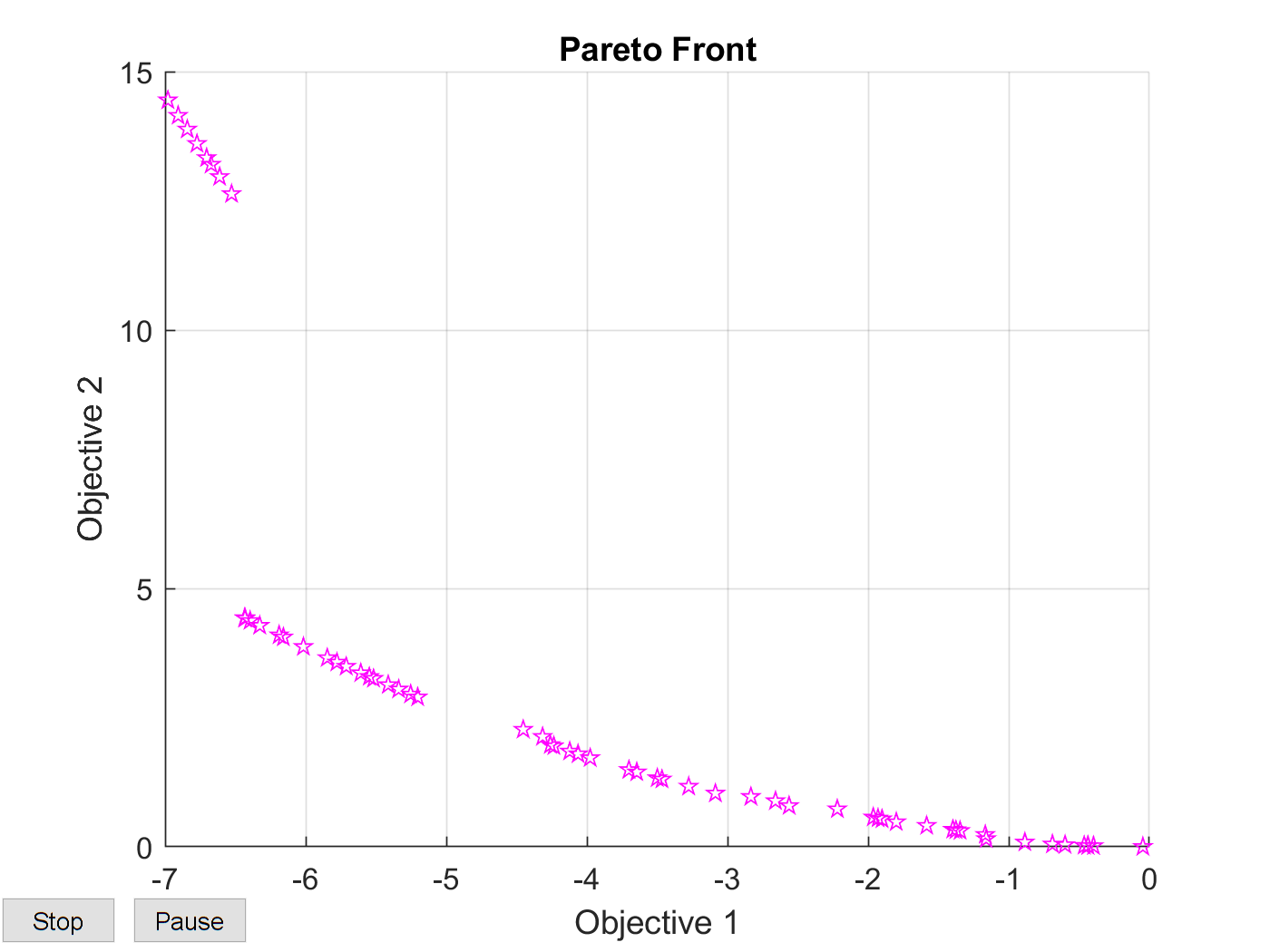```optionspareto = optimoptions('paretosearch','PlotFcn','psplotparetof')
funpareto = @(x) [-(beta(1,1)*x(1) + beta(2,1)*x(2) + beta(3,1)*x(3) + beta(4,1)*x(4) + beta(5,1)*x(5) + beta(6,1)*x(6) + beta(7,1)*x(7));
(((beta(1,1)*x(1) + beta(2,1)*x(2) + beta(3,1)*x(3) + beta(4,1)*x(4) + beta(5,1)*x(5) + beta(6,1)*x(6) + beta(7,1)*x(7)))^2)/(g*x((7))) ]
rng default % For reproducibility
x = paretosearch(funpareto,7,A,b,Aeq,beq,LB,UB,[],optionspareto);

funfgoalattain = @(x) [-(beta(1,1)*x(1) + beta(2,1)*x(2) + beta(3,1)*x(3) + beta(4,1)*x(4) + beta(5,1)*x(5) + beta(6,1)*x(6) + beta(7,1)*x(7));
(-((beta(1,1)*x(1) + beta(2,1)*x(2) + beta(3,1)*x(3) + beta(4,1)*x(4) + beta(5,1)*x(5) + beta(6,1)*x(6) + beta(7,1)*x(7)))^2)/(x((7)))];
goal = [1,1];
weight = [0.8,1];
xfgoalattain = fgoalattain(funfgoalattain,x0,goal,weight,A,b,Aeq,beq,LB,UB) ;
```

Having obtained the optimal values I modelled the geometry in Solidworks as a visual sanity check. I conducted a sensitivity analysis on the model to establish correlations between variables and how they affected the optimal value.

### Visual Sanity Check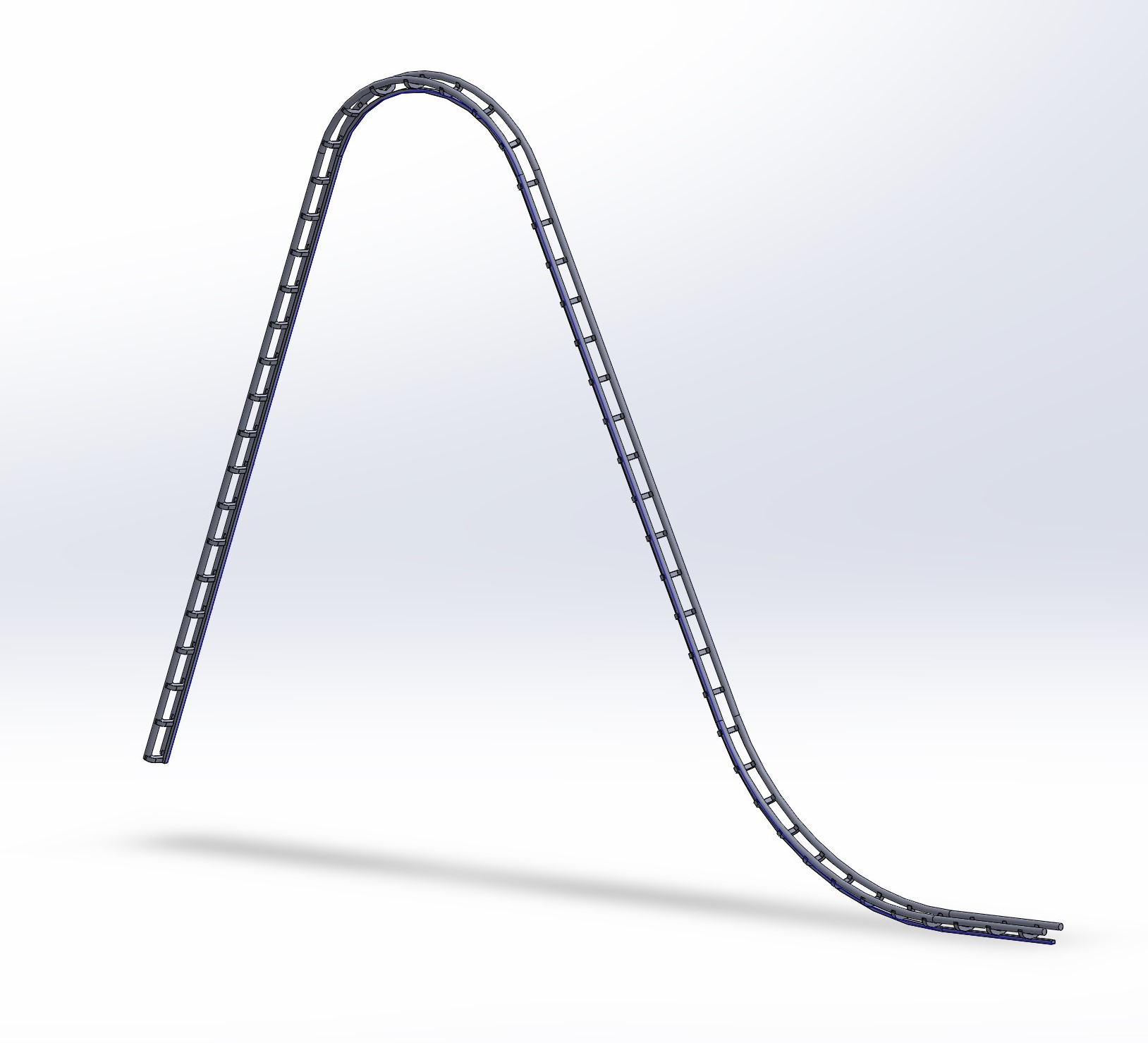### Report (my contribution is subsystem 2)